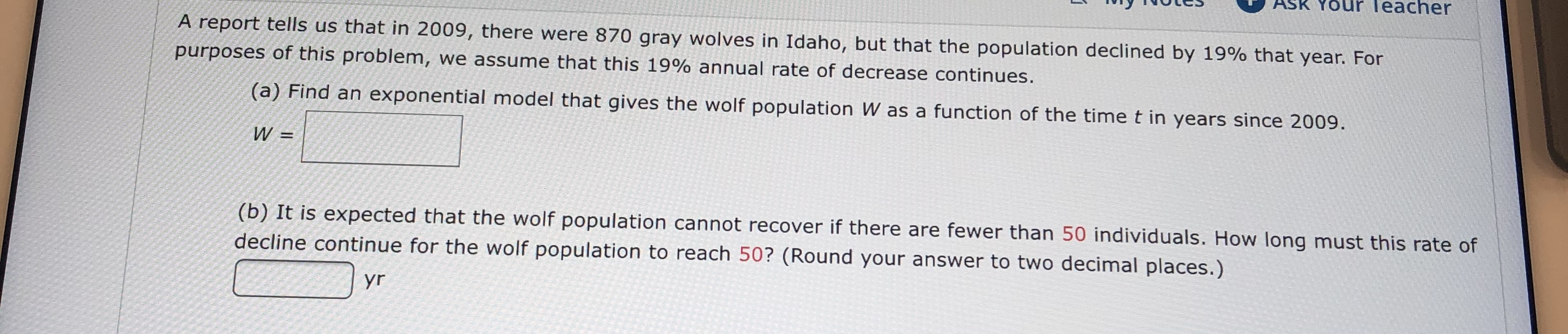# ASK YOUR Teacher A report tells us that in 2009, there were 870 gray wolves in Idaho, but that the population declined by 19% that year. For purposes of this problem, we assume that this 19% annual rate of decrease continues. (a) Find an exponential model that gives the wolf population W as a function of the time t in years since 2009 W = (b) It is expected that the wolf population cannot recover if there are fewer than 50 individuals. How long must this rate of decline continue for the wolf population to reach 50? (Round your answer to two decimal places.) yr

Questionhelp_outlineImage TranscriptioncloseASK YOUR Teacher A report tells us that in 2009, there were 870 gray wolves in Idaho, but that the population declined by 19% that year. For purposes of this problem, we assume that this 19% annual rate of decrease continues. (a) Find an exponential model that gives the wolf population W as a function of the time t in years since 2009 W = (b) It is expected that the wolf population cannot recover if there are fewer than 50 individuals. How long must this rate of decline continue for the wolf population to reach 50? (Round your answer to two decimal places.) yr fullscreen